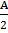# If the area of a square with side a is equal to the area of a triangle with base a, then the altitude of the triangle is   (a)(b) a (c) 2a (d) 4a

## Question ID - 50117 :- If the area of a square with side a is equal to the area of a triangle with base a, then the altitude of the triangle is   (a)(b) a (c) 2a (d) 4a

3537

Area of the square =Area of triangle =Next Question :

A practice is executing simple harmonic motion (SHM) of amplitude A, along the x-axis, about x=0.  When is potential Energy (PE) equals kinetic energy (KE), the position of the particle will be

 a.b.c.d. A